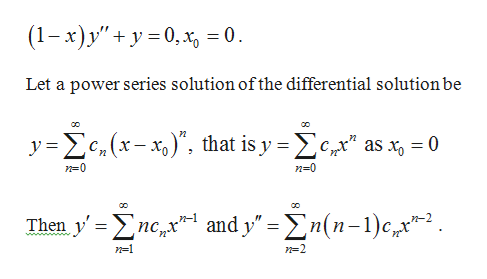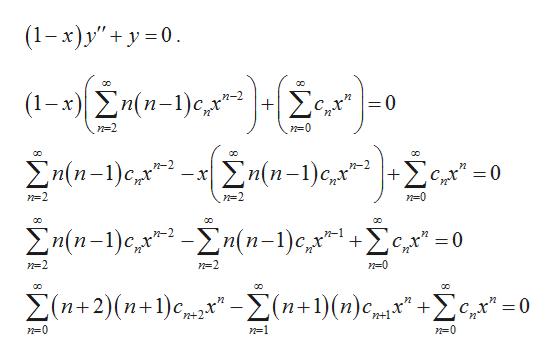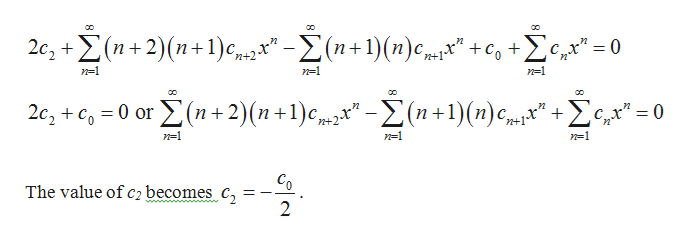# Given the differential equation:(1-x)y"+y=0, x0=0Find:Seek the power series solution for the differential equation about the given point x0; find the recurrence relationFind the first four terms in each of the two solutions, y1 and y2 (unless the series terminates sooner)By evaluating the Wronskian, W(y1,y2)(x0), show that y1 and y2 form a fundamental set of solutionsIf possible, find the general term in each solution

Question

Given the differential equation:

(1-x)y"+y=0, x0=0

Find:

• Seek the power series solution for the differential equation about the given point x0; find the recurrence relation
• Find the first four terms in each of the two solutions, y1 and y2 (unless the series terminates sooner)
• By evaluating the Wronskian, W(y1,y2)(x0), show that y1 and y2 form a fundamental set of solutions
• If possible, find the general term in each solution
check_circleExpert Solution
Step 1

Consider the differential equationhelp_outlineImage Transcriptionclose(1-х) у" + у -0, х, 3D0. Let a power series solution of the differential solution be y e(x-) that is y e as x 0 n 0 n-0 and y" (n-c Then y >n п-1 пс, х n-1 n-2 fullscreen
Step 2

Substitute y, y’ and y’’ inhelp_outlineImage Transcriptionclose(1-x)y"y0 n(n-1)cx e" | (1- 0 x n-2 n=0 Žn(n-1)e,x ]+£€,x". n(n-1)c 37 =0 C n=2 n-2 n(n-l)c (n-1)e,x 0 n-2 n-0 + _ n+1 n-0 n-1 n=0 fullscreen
Step 3

Thus, the two equation...help_outlineImage Transcriptioncloseco Σ. 2c, (n+2) (n+1) n+1)(n)c = 0 Cr n+1 n-1 n-1 n-1 2c, + c0 or(n+2)(n +1)e -(n +1) (n) cm" £" = 0 n-1 n=1 n=1 The value of c2 becomes c 2 fullscreen

### Want to see the full answer?

See Solution

#### Want to see this answer and more?

Solutions are written by subject experts who are available 24/7. Questions are typically answered within 1 hour*

See Solution
*Response times may vary by subject and question
Tagged in

### Math